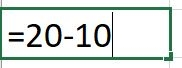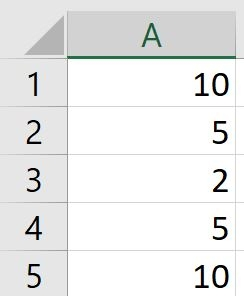Excel is a Microsoft spreadsheet application that can help with budgeting and accounts. It can do quite complex mathematical equations and formulas and offer visual mathematical data including graphs and pie charts.

You’ll need:

• A computer with Excel installed

Step 1: Subtract within a cell.

Simply enter a formula in a cell using the sign (-) and then press enter.The cell will appear as 10 as a result.

Step 2: Subtracting in a range

Adding a negative number is identical to subtracting one number from another. Use the SUM function to add negative numbers in a range. For example, SUM (100,-32,15,-6) returns 77.

Step 3: More subtracting formulasIf you want to minus the cells A1 and A5 for example, enter the formula A1-A5, then press enter on your keyboard. The cell will then change to '0'.

Have you looked at our other Excel guides that we have recommended below? For more formulas we advise looking at formulas and functions on the Office Support site.

This guide was last updated on 11/03/2019